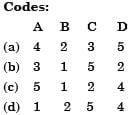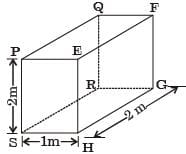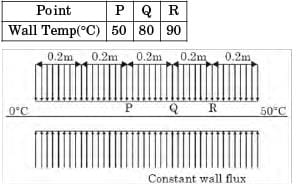GATE Past Year Questions: Free & Forced Convection

# GATE Past Year Questions: Free & Forced Convection Notes | Study Heat Transfer - Mechanical Engineering

## Document Description: GATE Past Year Questions: Free & Forced Convection for Mechanical Engineering 2022 is part of Forced Convection for Heat Transfer preparation. The notes and questions for GATE Past Year Questions: Free & Forced Convection have been prepared according to the Mechanical Engineering exam syllabus. Information about GATE Past Year Questions: Free & Forced Convection covers topics like and GATE Past Year Questions: Free & Forced Convection Example, for Mechanical Engineering 2022 Exam. Find important definitions, questions, notes, meanings, examples, exercises and tests below for GATE Past Year Questions: Free & Forced Convection.

Introduction of GATE Past Year Questions: Free & Forced Convection in English is available as part of our Heat Transfer for Mechanical Engineering & GATE Past Year Questions: Free & Forced Convection in Hindi for Heat Transfer course. Download more important topics related with Forced Convection, notes, lectures and mock test series for Mechanical Engineering Exam by signing up for free. Mechanical Engineering: GATE Past Year Questions: Free & Forced Convection Notes | Study Heat Transfer - Mechanical Engineering
 1 Crore+ students have signed up on EduRev. Have you?

Question for GATE Past Year Questions: Free & Forced Convection
Try yourself:Match List-I with List-ll and select the correct answer using the code given below the lists:
List-I
A. Grashof number
B. Schmidt number
C. Weber number
D. Fourier number

List-II
1. Mass diffusion
2. Transient heat conduction
3. Free convection
4. Forced convection
5. Surface tensionQuestion for GATE Past Year Questions: Free & Forced Convection
Try yourself:The ratio of momentum diffusivity (v) to thermal diffusivity (α), is called



Question for GATE Past Year Questions: Free & Forced Convection
Try yourself:Grashof number signifies the ratio of



Question for GATE Past Year Questions: Free & Forced Convection
Try yourself:In pool boiling the highest HTC occurs in



Question for GATE Past Year Questions: Free & Forced Convection
Try yourself:Heat transfer coefficients for free convection in gases, forced convection in gases and vapours, and for boiling water lie, respectively, in the range of



Question for GATE Past Year Questions: Free & Forced Convection
Try yourself:For the three-dimensional object shown in the figure below, five faces are insulated. The sixth face (PQRS), which is not insulated, interacts thermally with the ambient, with a convective heat transfer coefficient of 10W/m2K. The ambient temperature is 30°C. Heat is uniformly generated inside the object at the rate of 100 W/m3. Assuming the face PQRS to be at uniform temperature, its steady state temperature isQuestion for GATE Past Year Questions: Free & Forced Convection
Try yourself:Water (specifie heat, c = 4.18 kJ/kgK) enters a pipe at a rate 0.01 kg/s and a temperature of 20°C. The pipe, of diameter 50 mm and length 3 m, is subjected to a wall heat flux q"w in W/m2.

If q"w = 2500x, where x is in m and in the direction of flow (x = 0 at the inlet), the bulk means temperature of the water leaving the pipe in °C is



Question for GATE Past Year Questions: Free & Forced Convection
Try yourself:Water (specifie heat, c = 4.18 kJ/kgK) enters a pipe at a rate 0.01 kg/s and a temperature of 20°C. The pipe, of diameter 50 mm and length 3 m, is subjected to a wall heat flux q"w in W/m2.

If q"w = 5000 and the convection heat transfer coefficient at the pipe outlet is 1000 W/m2K, the temperature in °C at the inner surface of the pipe at the outlet is



Question for GATE Past Year Questions: Free & Forced Convection
Try yourself:For laminar forced convection over a flat plate, if the free stream velocity increases by a factor of 2, the average heat transfer coefficient​



Question for GATE Past Year Questions: Free & Forced Convection
Try yourself:The properties of mercury at 300 K are: Density = 13529 kg/m3, cp = 0.1393 kJ/kgK, dynamic viscosity = 0.1523 × 10–2 Ns/m2 and thermal conductivity = 8.540 W/mK. The Prandtl number of the mercury at 300 K is



Question for GATE Past Year Questions: Free & Forced Convection
Try yourself:In the laminar flow of air (Pr = 0.7) over a heated plate, if δ and δT denote, respectively, the hydrodynamic and thermal boundary layer thicknesses, then



Question for GATE Past Year Questions: Free & Forced Convection
Try yourself:For a hydrodynamically and thermally fully developed laminar flow through a circular pipe of constant cross-section. The Nusselt number at constant wall heat flux (Nuq) and that at constant wall temperature (NuT) are related as



Question for GATE Past Year Questions: Free & Forced Convection
Try yourself:The wall of a constant diameter pipe of length 1 m is heated uniformly with flux q” by wrapping a heater coil around it. The flow at the inlet to the pipe is hydrodynamically fully developed. The fluid is incompressible and the flow is assumed to be laminar and steady all through the pipe.
The bulk temperature of the fluid is equal to 0°C at the in let and 50°C at the exit. The wal l temperatures are measured at three locations, P, Q an d R as shown in the figure. The flow thermally develops after some distance from the inlet. The following measurements are made :Among the locations P, Q and R, the flow is thermally at :



The document GATE Past Year Questions: Free & Forced Convection Notes | Study Heat Transfer - Mechanical Engineering is a part of the Mechanical Engineering Course Heat Transfer.
All you need of Mechanical Engineering at this link: Mechanical Engineering

## Heat Transfer

58 videos|70 docs|85 tests
 Use Code STAYHOME200 and get INR 200 additional OFF

## Heat Transfer

58 videos|70 docs|85 tests

Track your progress, build streaks, highlight & save important lessons and more!

,

,

,

,

,

,

,

,

,

,

,

,

,

,

,

,

,

,

,

,

,

;